American Journal of Applied Mathematics
Volume 3, Issue 6, December 2015, Pages: 312-320

Enhancements of the Easter Algorithms (1940)

Charles Edward Ng’hwaya Masule

Institute of Mechanical Engineering, Mechanical Process Engineering and Environmental Technology, Dresden University of Technology, Dresden, Germany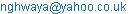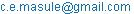Charles Edward Ng’hwaya Masule. Enhancements of the Easter Algorithms (1940). American Journal of Applied Mathematics. Vol. 3, No. 6, 2015, pp. 312-320. doi: 10.11648/j.ajam.20150306.21

Abstract: Easter is a Christian yearly festival which falls on a Sunday of a full moon on the 22nd March at the least. One question that had remained unanswered for years however is: why Easter does not fall on due Sundays of a full moon at times? In this publication, a reasonable way had been developed on how to interface the much known Oudin’s Easter algorithm  to enable the pulling of the date of Easter one day back such that the occurrence of Easter on a full moon is possible. This will mean that: since the lunar month is 29.5 days long , the age of a full moon is 14.75 days and that: since the age of a full moon in the Easter algorithm is taken to be 15 days, it makes sense to pull the date of Easter a day back if the 15th day of a new moon falls on a Monday because – algorithmically - 6 hours of the full moon fall on the Sunday.

Keywords: Easter Algorithm, Gregorian Calendar, Gauss Easter Algorithm, Metonic Cycle, Lunisolar Events, Sidereal Solar Event

Contents

1. Introduction

Easter is a lunisolar event like the Chinese New Year or the Hindu New year. The occurrence of these events is anchored to a sidereal solar event or an algorithmic solar event.

For Easter to take place in line with the ecclesiastical rules: a full moon must fall at the Vernal equinox on a Sunday at the least. The algorithmic Vernal equinox for Easter was set to be the 22nd of March .

Because the solar calendar makes about 6935 days after 19 solar years [1,2], and because the moon completes 235 moons plus about 2 days in the very same period of time [1,2], there exists a possibility of occurrences of a moon of the same age in every 19 years at any particular solar event, say the Vernal Equinox. On the Gregorian calendar, however, this fact is largely influenced by the system of leap years after the year 99 which means that a computation technique exploiting this material fact must incorporate correction factors with increasing years.

The Oudin’s Easter algorithm  exploits the occurrence of new moons in the 19-year cycle since the Vernal equinox of the year 0000 (fig. 1).

The weakness of the Oudin’s algorithm lies in that it cannot detect a due Sunday of a full moon like the Sunday of the 25th March 0001 and 28th March 2021 (fig. 2).

Contrary to the due Easter Sundays in fig. 2, the Oudin’s Easter algorithm sets the dates of Easter a week later: the 01st April 0001 and the 04th April 2021 (fig. 3).

Undocumented sources report that the Germany mathematician and physicist Johann Carl Friedrich Gauss had attempted with success to calculate the date of Easter without scribing figures on a piece of paper.

There exists a need to refine the Oudin’s Easter algorithm to enable Easter to fall on a due Sundays of a full moon.

2. Easter on the Gregorian Calendar

2.1. Comments to the Oudin’s Approach (1940)

Note: integer arithmetic is in action.

What is it about? The crucial part of the Easter algorithm is to understand that a lunar calendar is to be tracked beginning with the new moon of 22nd March 0000 (fig. A1 a). The primary objective is to calculate the number of days short to a full moon on the 22nd March of any particular Gregorian year.

Insider Secret: The Easter algorithm proceeds by using modal cycles called the 19-year cycle or Metonic cycle. A new moon is guaranteed after 19 years within ±2 days. Although there are deviations in occurrences of the new moon with increasing time lapse with respect to the reference cycle after the year 99 (fig. A1 a, A3, A4 and A5; table B1), any particular year must be fitted into the basic cycle in order to calculate Easter.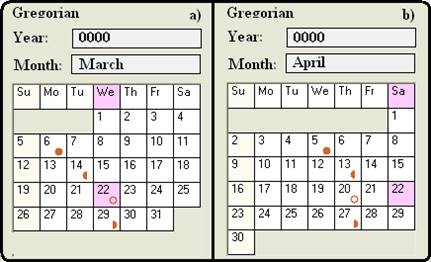Fig. 1. a) The Reference New Moon of 22nd of March 0000, b) the Full Moon of 05th April 0000; Moon Phases Algorithm Source: .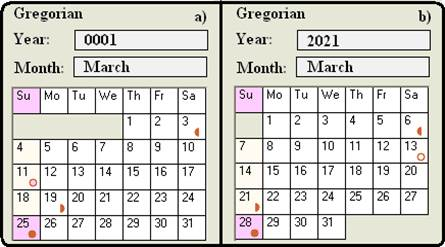Fig. 2. Due Sundays for Easter on Full Moons of 25th of March, 0001, and of 28th March 2021; Moon Phases Algorithm Source: .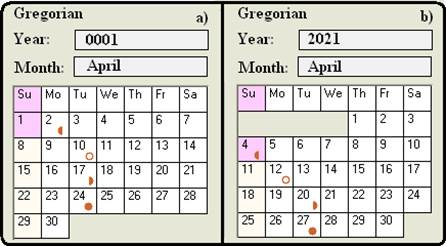Fig. 3. Easter Sundays from the Oudin’s algorithm on the 01st of April, 0001, and on the 04th April 2021; Moon Phases Algorithm Source: .

Warning: because a lunar year is 11 days short to the solar year, and that because 11 days plus 19 days makes 30 days (a lunar month is 29.5 days), one may think that 11 or 19 multiplied a the number of years n from a reference year gives out the age a new moon or the number of days short to the new moon, respectively, after the n years in the Easter algorithm. The answer is "No" for cross- Metonic cycles computations, because a lunar calendar needs a system of leap years .

Ecclesiastical Rules: Because Easter must fall on the algorithmic Vernal equinox (the 22nd of March) at the least and because Easter must fall on a Sunday of a full moon at the least , an opportunity offers itself to model the new moon of the 22nd March 0000 (fig. A1 a).

Without any efforts, the first Easter must fall on the 09th April 0000, the fourth day after the full moon (fig. A1 b).

The age of a New Moon after one Solar Year: The age of a new moon after one solar year will be approximately equal to 11 days because a lunar year is 11 days short to the solar year (fig. A2 a):

365.25 solar days - 354 lunar days = 11 days   (1)

The number of days short to the full moon on the 22nd of March 0001 comes out to be 3 (fig. A2 a).

So, n must be calculated first from the year y to get a slot n in the 19-year cycle :

n=y–19*(y div 19) which is equivalent to n = y mod 19  (2)

So, in a 19-year cycle, the number of days short to the full moon, i, is given by :

i = c - (c div 4) - ((c - k) div 3) + (19 * n) + 15   (3)

Now, for the year 0000, and 0001 (with c = 0; and k = 0 for now) the value of i counted from the 22nd of March is:

i = 15 for the year 0000 (4)

i = 34 for the year 0001 (5)

Because the length of a lunar is 29.5 days, the age of the lunar calculated above must be divided by 30 to have just the age of the new moon :

i = i mod 30   (6)

i = 15 for the year 0000 (fig. A1) (7)

i = 04 for the year 0001(fig. A2 a) (8)

Towards the Magic: It happens that the week day number for the day of the full moon of 05th April 0000 (Fig. A1: b) calculated in equation (9) is a Wednesday (with c being zero for now, i = 15, and y = 0) :

j = y + (y div 4) + i + 2 - c + (c div 4)    (9)

j = j - 7 * (j div 7) (equivalent to j = j mod 7) (10)

j = 3      (11)

It happens to be true that the week day numbers, j, out of equation (9) obey the standard numbers 0 to 6 for Sunday to Saturday respectively.

The Month Magic: It happens to be of great luck that the difference between i and j gives out a figure which is less than 4 for the case of Easter in March and greater or equal to 4 for the case of Easter in April :

l = i – j      (12)

For the year 0000 (with i = 15, and j = 3):

l = 12   (13)

For the year 0001 (with i = 4, and j = 0):

l = 4   (14)

Thus, calculating the month is ideal in the following equation :

m = 3 + (l + 40) div 44 (15)

For the year 0000 (with l = 12):

m = 4   (16)

For the year 0001 (with l = 4):

m = 4   (17)

The Date Magic: Now that the max of l is 3 for Easter to be in March and the length of March is 31 days, calculating the date is ideal as follows :

d = l + 28 - 31 * (m div 4) (18)

Only (l + 28) will determine the date of Easter in March, the rest of equation (18) will be zero.

For the year 0000 (with l = 12, m = 4):

d = 4   (19)

For the year 0001 (with l = 4, m = 4):

d = 1      (20)

The Influence of Centuries: As times lapses in terms of centuries there is a shift in the occurrence of new moons with respect to the 22nd of March at the beginning of the 19-year cycle in the Gregorian calendar (fig. A1 a, A3, A4, A5 and table B1) because of the reduced number of leap years. A similar situation will be experienced in the values of day week number, j. The century factor takes shape to affect i and j in equation (3) and (9) respectively, so:

c = y div 100   (21)

s = c - (c div 4) (22)

For the year 0000, c will have no effect:

For c = 0, s = 0   (23)

For c = 1, s = 1    (24)

For c = 2, s = 2 (25)

For c = 3, s = 3 (26)

For c = 4, s = 4 (27)

For c = 5, s = 4 (28)

Influence of the Reference: There is a factor k, anchored to the year 1700 which becomes significant when the year is 4200 (c = 42) and above:

k = (c - 17) div 25 (29)

z = (c - k) div 3      (30)

z will influence i and j in equation (3) and (9) respectively.

Oudin’s Algorithm in Summary :

c = y div 100   (31)

n = y – 19 * (y div 19) equivalent to n = y mod 19  (32)

k = (c – 17) div 25 (33)

i = c - (c div 4) - ((c - k) div 3) + (19 * n) + 15  (34)

i = i - 30 * (i div 30) equivalent to i = i mod 30  (35)

j = y + (y div 4) + i + 2 - c + (c div 4)  (36)

j = j - 7 * (j div 7) equivalent to j = j mod 7  (37)

l = i – j      (38)

m = 3 + (l + 40) div 44    (39)

d = l + 28 - 31 * (m div 4) (40)

2.2. Ng’hwaya’s Approach

Like in the Oudin’s approach  described in 1.1, this strategy sets the reference to be the 22nd March 0000, the day of the algorithmic Vernal Equinox of the year 0000 (fig. A1 a).

Unlike the Oudin’s approach, this approach will track the number of days lapsed since the 22nd March 0000, iSo, the number of days lapsed in complete 19-year cycles, iSo2, as well as the number of days in the running 19-year cycle, iSo3, for a particular Gregorian year yS.

It fits best to prepare the variables for adding the leap years as follows:

n1 = yS div 12     (41)

n2=yS mod12(equivalent to n2=yS - 12* (yS div 12))  (42)

n3 = n2 div 4    (43)

n5 = yS div 100   (44)

n6 = yS div 400   (45)

Whereas equation (41) helps to add all the leap days, equation (43) will add a leap day if the previous year is a leap year.

iSo is then gotten so:

iSo = 365 * yS + (n1 * 2) + (n1 * 1) + (n3) (46)

iSo = iSo - n5 (47)

iSo = iSo + n6 (48)

Equation (47) subtracts all the leap days in the years divisible by 100 which were included in the days lapsed iSo out of equation (46). Equation (48) adds back those leap days in the years divisible by 400.

In order to be able to track the days in a 19-year cycle, the number of such cycles lapsed, yS2, must be known and proceed so then:

yS2 = (yS div 19) * 19 (49)

n1 = yS2 div 12   (50)

n2 = yS2 mod 12    (51)

n3 = n2 div 4     (52)

n5 = yS2 div 100    (53)

n6 = yS2 div 400    (54)

The number of days lapsed in complete cycles, iSo2, is given by:

iSo2 = 365 * yS2 + (n1 * 2) + (n1 * 1) + (n3) (55)

iSo2 = iSo2 - n5    (56)

iSo2 = iSo2 + n6 (57)

The number of days iSo3 lapsed in the striking cycle is then given by:

iSo3 = iSo - iSo2 (58)

Because the 22nd March 0000 is a Wednesday and because iSo is 0 on that day, 0 is assigned as a week day number for that Wednesday which means that Sunday gets the number 4:

wSu = 4   (59)

Changing Reference of Week Day Number

At the beginning of every 19-year cycle the week day number will be given by:

wD = iSo2 mod 7 (60)

The week day number for Sunday changes so then:

wSu = (wSu - (wD - 0) + 7) mod 7 (61)

Getting the Full Moon

The approach of determining the number of days short to the full moon on the 22nd March is typical of the Oudin’s algorithm so provided  and explained in 1.1 above:

c = yS div 100   (62)

n = yS mod 19   (63)

k = (c - 17) div 25 (64)

iLu = c - (c div 4) - ((c - k) div 3) + (19 * n) + 15  (65)

iLu = iLu mod 30     (66)

Relative to the 19-year cycle, the age of the full moon is given by:

fLu = iSo3 + iLu      (67)

Getting to a Sunday

How many days should be added to get to a Sunday, eLu, the following equations provide:

delta = (wSu - fLu mod 7)    (68)

delta = (delta + 7) mod 7      (69)

eLu = fLu + delta     (70)

Getting the Month and Date of Easter

The difference between Easter, eLu and Vernal equinox, iSo3 will tell whether the corresponding moon was in March or April hence the date of Easter:

l = eLu - iSo3   (71)

m = 3 + (l + 40) div 50 (72)

d = l + 22 - 31 * (m div 4)    (73)

2.3. Easter on a Full Moon of Sunday or Monday

Because the Easter algorithm builds algorithmically on a day after the full moon in principle (see fig. A1 and equation (34) and (65)), getting Easter on a full moon can simply be achieved by pulling back Easter whenever a full moon shall algorithmically fall on a Monday. This can be realized by switching the week numbers to the standard of 0 to 6 for of Sunday to Saturday respectively. To start, the crude week number on the full moon is provided by wD2:

wD2 = (iSo + iLu) mod 7   (74)

Because the crude week number of the 22nd March 0000 is 0 (fig. A1), adding 3 to wD2 will standardize the number, so:

wS2 = 0 + (wD2 + 3) mod 7 (75)

Switching the week numbers of Sunday and Monday to 0 proceeds as follows:

Sel1 = 1 + (wS2 div 2) (76)

Sel2 = 1 + (Sel1 div 2) (77)

Sel3 = 1 + (Sel2 div 2) (78)

Sel4 = Sel3 div 2   (79)

Because the selector Sel4 takes the values of 0 for Sunday and Monday and 1 otherwise, the pushing of Easter to a Sunday that follows will be prevented in equation (81):

delta = (wSu - fLu mod 7) (80)

delta = (delta + (7 * Sel4)) mod 7 (81)

eLu = fLu + delta   (82)

The computation of the month and date of Easter proceeds so then:

l = eLu - iSo3   (83)

m = 3 + (l + 40) div 50    (84)

d = l + 22 - 31 * (m div 4)    (85)

3. Discussion

There are four aspects that play part in the Easter algorithm: linking the lunar calendar to the solar calendar, tracking of the lunar calendar, the global days counter, the local Metonic days counter, and the local post-Equinox days counter:

Linking the Lunar to the Solar Calendars: Somewhat similar to the Hebrew lunisolar calendar, the Chinese lunisolar calendar, and the Newcomb’s moon phases algorithm, synchronizing the lunar calendar to the solar calendar is primary to the Easter algorithm. This date is the 22nd of March 0000 on the Gregorian calendar .

Tracking of the Lunar Calendar: Because the lunar year is 11 days shorter to the solar year, the age of a moon and the number of days short to the next new moon on the 22nd of March starting from the 22nd of March 0000 create series which enables the tracking of the lunar calendar. Whereas the ages provide the series: 0, 11, 22, 33, 44, 55, 66, 77, 88, . . . , the numbers of days short to the new moon provide the series: 0, 19, 38, 57, 76, 95, 114, 133, 152, . . . for "n x 11" and "n x 19" respectively (fig. A1 and A2). Whereas the 11-series works backwards, the 19-series works forwards.

Although the number of days short to the new moon is soaring with increasing number of years in the Metonic cycle, n, what counts is the remainder of that number when divided by 30 (the lunar length in days):

i = (n*19) mod 30    (86)

15 must be added in equation (86) to get to a full moon:

i = (15 + (n*19)) mod 30 (87)

Contrary to the effect attained in equation (46), the leap days in the years divisible by 100 must be added, whereas those leap days in the years divisible by 400 must be subtracted by adding "c - (c div 4) - ((c - k) div 3)":

i = c-(c div 4) - ((c - k) div 3) + (19 * n) + 15 (88)

i = i mod 30  (89)

Global Days Counting: This parameter will track the number of days lapsed since the 22nd of March 0000. This parameter is indispensable if the week day number of any particular day is to be determined counting from the reference. The "iSo" out of equation (48) is easily converted to a week day number using the modulo-operator (week day number = iSo mod 7). It won’t be possible to determine Easter falling on a full moon without this parameter. Standardizing this parameter to the Oudin’s week day number proceeds as follows:

j = (iSo + 3) mod 7     (90)

j = j – 1       (91)

Equation (90) will standardize the week day number to 0 to 6 for Sunday to Saturday, respectively, because the reference has a zero and is a Wednesday. Equation (91) will synchronize the value of j to the Oudin’s value of j. The jinx of the Oudin’s equation (36) is exactly here: the Oudin’s technique adds 2 instead of 3 to make things work in the subsequent steps of determining the date and month of Easter. The Oudin’s technique can be said to be holistic from this step onwards. And, because of this holistic approach, the Oudin’s algorithm will fail to catch Easter on a full moon, although the week day number, j, already has got the needed clue of a Sunday or Monday of a full moon. The Oudin’s technique will only succeed with a major overhaul.

But, to give the Devil his dues:

365 mod 7 = 1     (92)

365 * 2 mod 7 = 2 (93)

365 * 6 mod 7 = 6 (94)

365 * 7 mod 7 = 0 (95)

It means that the need to track the number of days from the reference to get the week day number at the full moon does not arise for that "Devil". And, because the solar year is about 365.25 days long, a day shall complete after every 4 years, which means that the following holds for the years between 0 and 99 when c is zero:

j = y + (y div 4) + i + 2  (96)

j = j mod 7      (97)

Adding 2 in equation (92) is an acceptable modeling technique to get to the desired end.

With the intrusion of the century, the number of leap years gets lesser which means they should be subtracted. But, the ones divisible by 400 are leap years and therefore must be added back:

j = y + (y div 4) + i + 2 - c + (c div 4) (98)

j = j mod 7    (99)

Local Metonic Days Counting: This parameter will track the number of days lapsed since the first 22nd of March in the running Metonic cycle.

Local Post-Equinox Days Counting: This parameter will track the number of days lapsed since the 22nd of March in the year of interest.

4. Conclusion

The Ng’hwaya’s algorithm has proven to bring about the revolution in the computation of Easter in that the occurrence of Easter on a full moon is possible. For any Gregorian year yS, the Easter month m and the Easter date d can be calculated as follows:

n1 = yS div 12   (100)

n2 = yS mod 12 (101)

n3 = n2 div 4   (102)

n5 = yS div 100 (103)

n6 = yS div 400 (104)

iSo = 365 * yS + (n1 * 2) + (n1 * 1) + (n3) (105)

iSo = iSo - n5    (106)

iSo = iSo + n6   (107)

yS2 = (yS div 19) * 19 (108)

n1 = yS2 div 12 (109)

n2 = yS2 mod 12 (110)

n3 = n2 div 4    (111)

n5 = yS2 div 100 (112)

n6 = yS2 div 400   (113)

iSo2 = 365 * yS2 + (n1 * 2) + (n1 * 1) + (n3) (114)

iSo2 = iSo2 - n5   (115)

iSo2 = iSo2 + n6   (116)

iSo3 = iSo - iSo2   (117)

wSu = 4   (118)

wD = iSo2 mod 7    (119)

wSu = (wSu - (wD - 0) + 7) mod 7 (120)

c = yS div 100      (121)

n = yS mod 19    (122)

k = (c - 17) div 25    (123)

iLu = c - (c div 4) - ((c - k) div 3) + (19 * n) + 15  (124)

iLu = iLu mod 30     (125)

fLu = iSo3 + iLu     (126)

wD2 = (iSo + iLu) mod 7    (127)

wS2 = 0 + (wD2 + 3) mod 7    (128)

Sel1 = 1 + (wS2 div 2)    (129)

Sel2 = 1 + (Sel1 div 2)      (130)

Sel3 = 1 + (Sel2 div 2)    (131)

Sel4 = Sel3 div 2       (132)

delta = (wSu - fLu mod 7)    (133)

delta = (delta + (7 * Sel4)) mod 7 (134)

eLu = fLu + delta   (135)

l = eLu - iSo3   (136)

m = 3 + (l + 40) div 50   (137)

d = l + 22 - 31 * (m div 4)      (138)

A more simplified version of the Ng’hwaya’s algorithm proceeds as follows:

n1 = yS div 12    (139)

n2 = yS mod 12 (140)

n3 = n2 div 4    (141)

n5 = yS div 100 (142)

n6 = yS div 400 (143)

iSo = 365 * yS + (n1 * 2) + (n1 * 1) + (n3) (144)

iSo = iSo - n5      (145)

iSo = iSo + n6      (146)

c = yS div 100        (147)

n = yS mod 19    (148)

k = (c - 17) div 25    (149)

iLu = c - (c div 4) - ((c - k) div 3) + (19 * n) +15  (150)

iLu = iLu mod 30    (151)

iSo = (iSo + iLu + 2) mod 7    (152)

Sel1 = 1 + (iSo div 2)    (153)

Sel2 = 1 + (Sel1 div 2)    (154)

Sel3 = 1 + (Sel2 div 2)    (155)

Sel4 = Sel3 div 2    (156)

delta = (7 - iSo)    (157)

delta = (delta + (7 * Sel4)) mod 7 (158)

iSu = iLu + delta – 1    (159)

m = 3 + (iSu + 40) div 50    (160)

d = iSu + 22 - 31 * (m div 4) (161)

The overhauled Oudin’s technique can now have a new hair cut:

c = y div 100      (162)

n = y – 19 * (y div 19) equivalent to n = y mod 19 (163)

k = (c – 17) div 25 (164)

i = c - (c div 4) - ((c - k) div 3) + (19 * n) + 15 (165)

i = i - 30 * (i div 30) equivalent to i = i mod 30  (166)

j = y + (y div 4) + i + 2 - c + (c div 4)    (167)

j = j - 7 * (j div 7) equivalent to j = j mod 7  (168)

Sel1 = 1 + (j div 2)    (169)

Sel2 = 1 + (Sel1 div 2)    (170)

Sel3 = 1 + (Sel2 div 2)    (171)

Sel4 = Sel3 div 2    (172)

delta = (7 - j)      (173)

delta = (delta + (7 * Sel4)) mod 7 (174)

iSu = i + delta – 1    (175)

m = 3 + (iSu + 40) div 50    (176)

d = iSu + 22 - 31 * (m div 4) (177)

Appendix A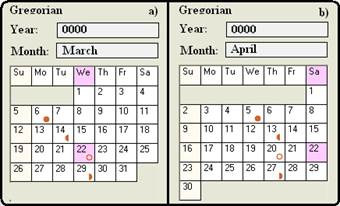Fig. A1. a) The Reference New Moon of 22nd of March 0000, b) The Full Moon of 05th April 0000; Moon Phases Algorithm Source: .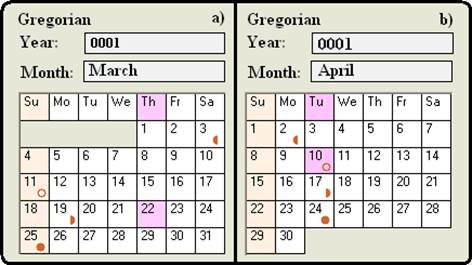Fig. A2. a) A New Moon 11 Days Old on the 22nd of March, 0001. b) the Next New Moon 19 days ahead; Moon Phases Algorithm Source: .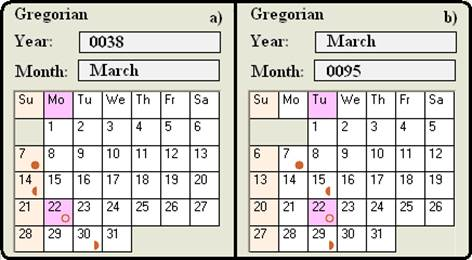Fig. A3. a) A New Moon on the 22nd of March, 0038, b) A New Moon on the 22nd of March, 0095; Moon Phases Algorithm Source: .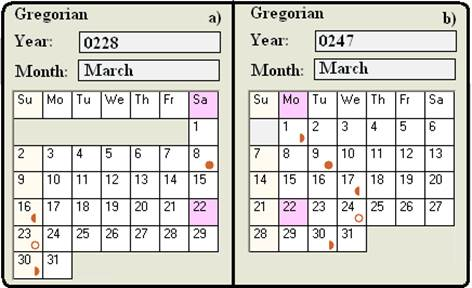Fig. A4. a) A New Moon on the 23rd of March, 0228, b) A New Moon on the 24th of March, 0247; Moon Phases Algorithm Source: .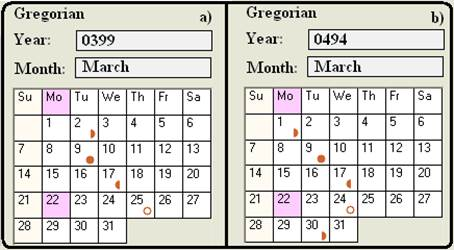Fig. A5. a) A New Moon on the 25th of March, 0399, b) A New Moon on the 24th of March, 0494; Moon Phases Algorithm Source: .

Appendix B

Table B1. Increasing Time Lapse v's New Moons of March .

 Year (=19xn) New Moon 0000 22-March 0011 23-March 0019 23-March 0228 23-March 0247 24-March 0342 24-March 0494 24-March 0513 25-March 0532 24-March 0551 25-March 0570 24-March 0589 24-March 0608 24-March 0627 26-March 0646 25-March 0665 25-March 0684 24-March 0703 26-March 0722 25-March 0741 25-March 0760 25-March 0779 26-March 0798 26-March 0817 25-March 0836 25-March 0855 25-March 0874 25-March 0893 25-March 0912 26-March 0931 27-March 0950 26-March 0969 25-March 0988 25-March 1007 27-March 1026 27-March 1045 27-March 1064 26-March 1083 27-March 1102 27-March 1121 27-March 1406 29-March 1615 29-March 1805 30-March 1995 31-March 2014 30-March

References

1. Seidelmann, P. K. (ed.): Explanatory Supplement to the Astronomical Almanac, Chapter 12, "Calendars", by L. E. Doggett, ISBN 0-935702-68-7, (University Science Books, CA, 1992)
2. Perry, R.H. and Green, D.W.: Perry's Chemical Engineers' Handbook, 8th Edition, (McGraw-Hill, 2007)
3. Meeus J.: Astronmical Algorithms, 2nd Edition, (Willmann-Bell, 1999)
4. NASA Computational Case Study: Where Is My Moon? Comput. Sci. Eng. 16, 92 (2014)
5. Oostra, B.: Introducing the Moon's Orbital Eccentricity, Phys. Teach. 52, 460 (2014)
6. Noordeh, E., Hall, P. and Cuk, M.: Simulating the Phases of the Moon Shortly After Its Formation, Phys. Teach. 52, 39 (2014)
7. Bates, A.: Galilean Moons, Kepler's Third Law, and the Mass of Jupiter, Phys. Teach. 51, 428 (2013)
8. Eisenstaedt, J.: From Newton to Einstein: A forgotten relativistic optics of moving bodies, Am. J. Phys. 75, 741 (2007)
9. McCall, M.: Gravitational orbits in one dimension, Am. J. Phys. 74, 1115 (2006)
10. Hussain, Z.: On Newton's Law of Attractions, Am. J. Phys. 19, 146 (1951).

 Contents 1. 2. 2.1. 2.2. 2.3. 3. 4.
Article ToolsAbstractPDF(2245K)